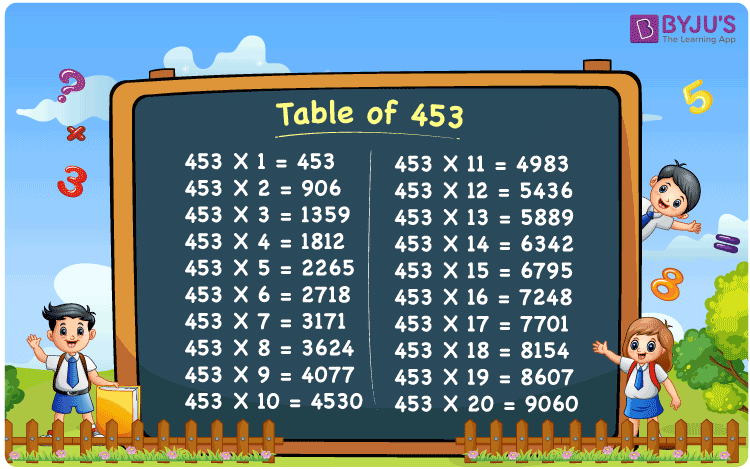Checkout JEE MAINS 2022 Question Paper Analysis : Checkout JEE MAINS 2022 Question Paper Analysis :

# Table of 453

The Table of 453 is given here in tabular format to help students learn the multiples of 453. Students can also download the multiplication table of 453 in PDF format for 20 times here. This table is very helpful to calculate problems in a quick manner based on the 453 times table. Multiplication tables help students to calculate by mind quickly, which is vital for appearing in other competitive exams.## What is the 453 Times Table?

453 times table is nothing but the multiplication table of 453. This table represents the multiples of 453 when we multiply the number 453 with any natural number. The table of 453 can be written by multiplication and by adding 453 repeatedly. The below chart helps students to learn the multiplication table of 453 in multiplication as well as in addition form.

 453×1 = 453 453 453×2 = 906 453 + 453 = 906 453×3 = 1359 453 + 453 + 453 = 1359 453×4 = 1812 453 + 453 + 453 + 453 = 1812 453×5 = 2265 453 + 453 + 453 + 453 + 453 = 2265 453×6 = 2718 453 + 453 + 453 + 453 + 453 + 453 = 2718 453×7 = 3171 453 + 453 + 453 + 453 + 453 + 453 + 453 = 3171 453×8 = 3624 453 + 453 + 453 + 453 + 453 + 453 + 453 + 453 = 3624 453×9 = 4077 453 + 453 + 453 + 453 + 453 + 453 + 453 + 453 + 453 = 4077 453×10 = 4530 453 + 453 + 453 + 453 + 453 + 453 + 453 + 453 + 453 + 453 = 4530

## Multiplication Table of 453

The multiplication table of 453 up to 20 multiples given below helps students to solve all the textbook problems with ease without any reference material.

 453 × 1 = 453 453 × 2 = 906 453 × 3 = 1359 453 × 4 = 1812 453 × 5 = 2265 453 × 6 = 2718 453 × 7 = 3171 453 × 8 = 3624 453 × 9 = 4077 453 × 10 = 4530 453 × 11 = 4983 453 × 12 = 5436 453 × 13 = 5889 453 × 14 = 6342 453 × 15 = 6795 453 × 16 = 7248 453 × 17 = 7701 453 × 18 = 8154 453 × 19 = 8607 453 × 20 = 9060

## Solved Example on the Table of 453

Example 1:

Calculate the difference between two consecutive multiples of 453.

Solution:

The difference between two consecutive multiples of 453 is 453.

For example: 453 x 9 = 4077 and 453 x 10 = 4530.

4530 – 4077 = 453.

## Frequently Asked Questions on Table of 453

### What is the sum of factors of 453?

The factors of 453 are 1, 3, 151 and 453. Sum = 1 + 3 + 151 + 453 = 608. Therefore, sum of factors of 453 is 608.

### Evaluate 453 times 15 minus 453 times 11 plus 8.

453 times 15 = 453 x 15 = 6795. 453 times 11 = 453 x 11 = 4983. 453 times 15 – 453 x 11 plus 8 = 6795 – 4983 + 8 = 1820.

### What is the value of 19 times 453?

19 times 453 is equal to 8607.